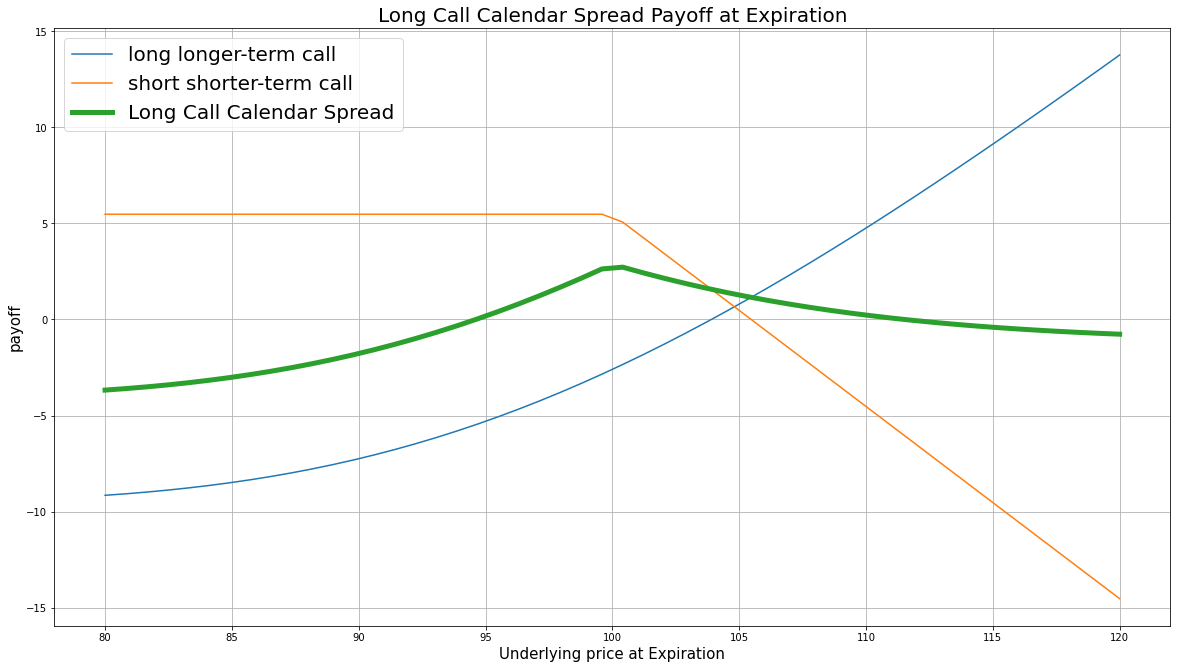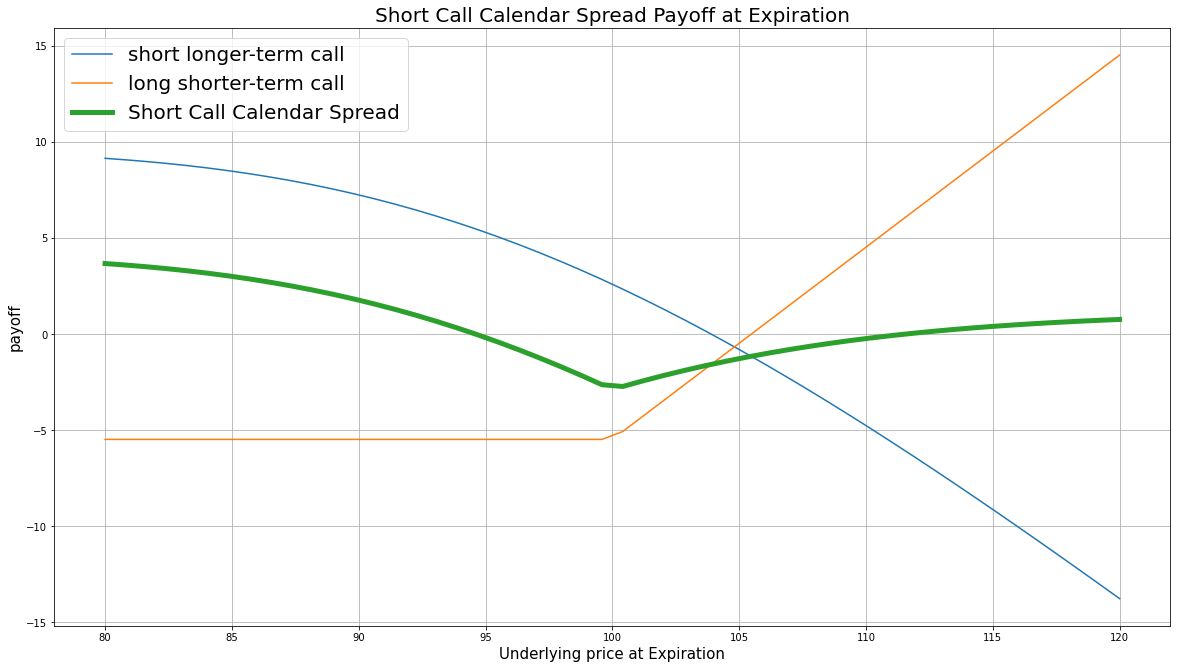# Option Strategies

### Introduction

Call calendar spread, also known as call horizontal spread, is a combination of a longer-term (far-leg/front-month) call and a shorter-term (near-leg/back-month) call, where all calls have the same underlying stock and the same strike price. The call calendar spread can be long or short.

The long call calendar spread consists of buying a longer-term call and selling a shorter-term call. This strategy profits from a decrease in the underlying price. It also profits from the time decay value because the theta $\theta$ (the Option price decay by 1 day closer to maturity) of the shorter-term call is larger than longer-term call.

The short call calendar spread consists of selling a longer-term call and buying a shorter-term call. The strategy profits from from an increase in the underlying price.

### Implementation

1. In the Initialize method, set the start date, end date, cash, and Option universe.
2. private Symbol _symbol;

public override void Initialize()
{
SetStartDate(2017, 2, 1);
SetEndDate(2017, 2, 19);
SetCash(500000);

_symbol = option.Symbol;
option.SetFilter(universe => universe.IncludeWeeklys()
.Strikes(-1, 1)
.Expiration(TimeSpan.FromDays(0), TimeSpan.FromDays(62)));
}
def Initialize(self) -> None:
self.SetStartDate(2017, 2, 1)
self.SetEndDate(2017, 2, 19)
self.SetCash(500000)

self.symbol = option.Symbol
option.SetFilter(self.UniverseFunc)

def UniverseFunc(self, universe: OptionFilterUniverse) -> OptionFilterUniverse:
return universe.Strikes(-1, 1).Expiration(timedelta(0), timedelta(62))
3. In the OnData method, select the expiration and strikes of the contracts in the strategy legs.
4. public override void OnData(Slice slice)
{
if (Portfolio.Invested) return;

// Get the OptionChain
var chain = slice.OptionChains.get(_symbol, null);
if (chain == null || chain.Count() == 0) return;

// Get the ATM strike
var atmStrike = chain.OrderBy(x => Math.Abs(x.Strike - chain.Underlying.Price)).First().Strike;

// Select the ATM call Option contracts
var calls = chain.Where(x => x.Strike == atmStrike && x.Right == OptionRight.Call);
if (calls.Count() == 0) return;

// Select the near and far expiry dates
var expiries = calls.Select(x => x.Expiry).OrderBy(x => x);
var nearExpiry = expiries.First();
var farExpiry = expiries.Last();
def OnData(self, slice: Slice) -> None:
if self.Portfolio.Invested: return

# Get the OptionChain
chain = slice.OptionChains.get(self.symbol, None)
if not chain: return

# Get the ATM strike
atm_strike = sorted(chain, key=lambda x: abs(x.Strike - chain.Underlying.Price)).Strike

# Select the ATM call Option contracts
calls = [i for i in chain if i.Strike == atm_strike and i.Right == OptionRight.Call]
if len(calls) == 0: return

# Select the near and far expiry dates
expiries = sorted([x.Expiry for x in calls])
near_expiry = expiries
far_expiry = expiries[-1]
5. In the OnData method, call the OptionStrategies.CallCalendarSpread method and then submit the order.
6. var optionStrategy = OptionStrategies.CallCalendarSpread(_symbol, atmStrike, nearExpiry, farExpiry);
Sell(optionStrategy, 1);     // if short call calendar spread
option_strategy = OptionStrategies.CallCalendarSpread(self.symbol, atm_strike, near_expiry, far_expiry)
self.Sell(option_strategy, 1)   # if short call calendar spread

Option strategies synchronously execute by default. To asynchronously execute Option strategies, set the asynchronous argument to Falsefalse. You can also provide a tag and order properties to the Buy and Sell methods.

Buy(optionStrategy, quantity, asynchronous, tag, orderProperties);
Sell(optionStrategy, quantity, asynchronous, tag, orderProperties);

self.Buy(option_strategy, quantity, asynchronous, tag, order_properties)
self.Sell(option_strategy, quantity, asynchronous, tag, order_properties)


### Strategy Payoff

The call calendar spread can be long or short.

The long call calendar spread is a limited-reward-limited-risk strategy. The payoff at the shorter-term expiration is

$$\begin{array}{rcll} C^{\textrm{short-term}}_T & = & (S_T - K)^{+}\\ P_T & = & (C^{\textrm{long-term}}_T - C^{\textrm{short-term}}_T + C^{\textrm{short-term}}_0 - C^{\textrm{long-term}}_0)\times m - fee\\ \end{array}$$ $$\begin{array}{rcll} \textrm{where} & C^{\textrm{short-term}}_T & = & \textrm{Shorter term call value at time T}\\ & C^{\textrm{long-term}}_T & = & \textrm{Longer term call value at time T}\\ & S_T & = & \textrm{Underlying asset price at time T}\\ & K & = & \textrm{Strike price}\\ & P_T & = & \textrm{Payout total at time T}\\ & C^{\textrm{short-term}}_0 & = & \textrm{Shorter term call value at position opening (credit received)}\\ & C^{\textrm{long-term}}_0 & = & \textrm{Longer term call value at position opening (debit paid)}\\ & m & = & \textrm{Contract multiplier}\\ & T & = & \textrm{Time of shorter term call expiration} \end{array}$$

The following chart shows the payoff at expiration:The maximum profit is undetermined because it depends on the underlying volatility. It occurs when $S_T = S_0$ and the spread of the calls are at their maximum.

The maximum loss is the net debit paid, $C^{\textrm{short-term}}_0 - C^{\textrm{long-term}}_0$. It occurs when the underlying price moves very deep ITM or OTM so the values of both calls are close to zero.

If the Option is American Option, there is risk of early assignment on the sold contract. If the buyer exercises the call you sell, you could lose all the debit you received if you don't close the long call and the underlying price drops below the long call strike price.

The short call calendar spread is a limited-reward-limited-risk strategy. The payoff at the shorter-term expiration is

$$\begin{array}{rcll} C^{\textrm{short-term}}_T & = & (S_T - K)^{+}\\ P_T & = & (C^{\textrm{short-term}}_T - C^{\textrm{long-term}}_T + C^{\textrm{long-term}}_0 - C^{\textrm{short-term}}_0)\times m - fee \end{array}$$ $$\begin{array}{rcll} \textrm{where} & C^{\textrm{short-term}}_T & = & \textrm{Shorter term call value at time T}\\ & C^{\textrm{long-term}}_T & = & \textrm{Longer term call value at time T}\\ & S_T & = & \textrm{Underlying asset price at time T}\\ & K & = & \textrm{Strike price}\\ & P_T & = & \textrm{Payout total at time T}\\ & C^{\textrm{short-term}}_0 & = & \textrm{Shorter term call value at position opening (debit paid)}\\ & C^{\textrm{long-term}}_0 & = & \textrm{Longer term call value at position opening (credit received)}\\ & m & = & \textrm{Contract multiplier}\\ & T & = & \textrm{Time of shorter term call expiration} \end{array}$$

The following chart shows the payoff at expiration:The maximum profit is the net credit received, $C^{\textrm{long-term}}_0 - C^{\textrm{short-term}}_0$. It occurs when the underlying price moves very deep ITM or OTM so the values of both calls are close to zero.

The maximum loss is undetermined because it depends on the underlying volatility. It occurs when $S_T = S_0$ and the spread of the 2 calls are at their maximum.

If the Option is American Option, there is risk of early assignment on the sold contract. If you don't close the call positions together, the naked short call will have unlimited drawdown risk after the long call expires.

### Example

The following table shows the price details of the assets in the long version algorithm:

AssetPrice ($)Strike ($)
Longer-term call at the start of the trade4.40835.00
Shorter-term call at the start of the trade36.80767.50
Longer-term call at time $T$31.35835.00
Underlying Equity at time $T$829.08-

Therefore, the payoff at time $T$ (the expiration of the short-term call) is

$$\begin{array}{rcll} C^{\textrm{short-term}}_T & = & (S_T - K)^{+}\\ & = & (828.07-800.00)^{+}\\ & = & 28.07\\ P_T & = & (C^{\textrm{long-term}}_T - C^{\textrm{short-term}}_T + C^{\textrm{short-term}}_0 - C^{\textrm{long-term}}_0)\times m - fee\\ & = & (31.35-28.07+11.30-20.00)\times100-1.00\times2\\ & = & -544 \end{array}$$

So, the strategy losses \$544.

The following algorithm implements a long call calendar spread Option strategy:

You can also see our Videos. You can also get in touch with us via Discord.